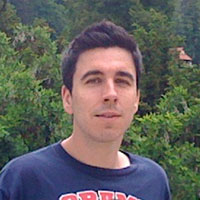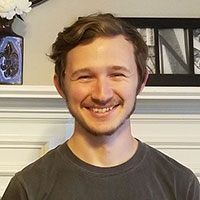# Mathematics

### Hadamard Matrices, Secret Codes, Concert Halls and Quantum Teleportation Schemes

We will start the course with a rigorous introduction to logic and proof writing. Various types of proofs will be presented: direct proofs, non-constructive proofs, proofs by contradiction and proofs by induction. We will also review some important number theory results, involving prime numbers and modular arithmetic.We will then discuss matrix theory. In mathematics, a matrix is a rectangular table of numbers arranged in rows and columns. Matrices can be added and multiplied, just like numbers. However, matrices can also behave very differently from numbers. For instance, matrices do not commute when multiplied, meaning that A times B may not equal B times A. We will study matrices and their applications to computer graphics, the ranking of web pages in search engines, and quantum mechanics.

In the last part of the course we will focus on a special class of matrices called Hadamard matrices. These are matrices having n rows and n columns and all entries equal to 1 or -1, such that each two distinct rows agree on exactly half positions (and disagree on the other half). It is easy to draw them by representing the 1 entries as white squares, and the -1 entries as a black squares.

The definition of a Hadamard matrix is deceptively simple, but it is not known for which n’s such matrices exist. The famous Hadamard conjecture asserts that for every integer n divisible by 4 there exists a Hadamard matrix of size n, but nobody has managed to prove this 150-year old conjecture.

We will use our number theory and matrix knowledge to investigate different ways to construct Hadamard matrices. We will also present applications to coding theory, acoustics, and quantum teleportation.

#### Student Learning Outcomes

Upon successful completion of this course, a student will be able to:

• Apply the logical structure of proofs and work symbolically with connectives and quantifiers to produce logically valid, correct and clear arguments.
• Perform set operations on finite and infinite collections of sets and be familiar with properties of set operations.
• Write solutions to problems and proofs of theorems that meet rigorous standards based on content, organization and coherence, argument and support, and style and mechanics.
• Describe the real line as a complete, ordered field.
• Check if a set with two given binary operations is a ring or a Give examples and non-examples of rings and fields (including examples from matrix theory and from modular arithmetic).
• Construct direct and indirect proofs and proofs by induction and determinethe appropriateness of each type in a particular setting.
• Master the concepts of divisibility, congruence, greatest common divisor, prime, prime- factorization, and quadratic reciprocity.
• Master matrix algebra concepts such as matrices, vectors, and linear transformations.
• Be familiar with the definition, properties and current state of classification of Hadamard matrices.
• Formulate and prove conjectures about numerical patterns in number theory and matrix algebra.

#### Course Requirements/Examinations

The criteria for assigning grades for the course are the following:

• Completion of weekly homework (60% of the grade)
• Class participation (20% of the grade)
• Team-based preparation of a poster/presentation (20% of the grade)

Credit Hours: 3

#### 2020 Math Research

Click on the links below to view the research posters of the 2020 GSSE Mathematics Scholars.

Matrices and their Applications

The Fourth Dimension and Beyond

Famous Statements in Number Theory

### Dr. Remus Nicoara, Course Director

#### Dr. Remus Nicoara, Course DirectorDr. Remus Nicoara earned his Ph.D. in Mathematics from UCLA, and his Bachelor’s Degree from the University of Bucharest, Romania. He is currently a Professor of Mathematics and Director of the Math Honors Program at the University of Tennessee. His main research interest lies in von Neumann algebras, which are algebras of operators that model quantum mechanical systems. Outside of work, Remus likes to hike, bike and garden while thinking about math. He enjoys meditation, Sci-Fi books, and Hanayama puzzles. He is also an avid gamer and he currently teaches a class about video games and math, called Math Effect.

### Mike Hanson, Teaching Assistant

#### Mike Hanson, Teaching AssistantMike is a graduate student in mathematics at the University of Tennessee studying Number Theory. After graduating, he plans to continue his career in academia as a researcher in mathematics. Aside from math, Mike enjoys making and listening to music, drawing, and traveling. He is very excited to assist the Governor’s school math students while they learn about proofs, number theory and matrices.